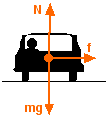# Static friction

vitesse86

## Homework Statement

I have a general question about circular motion of a car on a flat curve.
What must the static friction koefficient be if the car can make the swing by 60km/h?

Thank you,

## Homework Equations

- We have to follow newtons 2nd law.

- Fcentripetal = m*(v^2/r)

known stats:
r= 150m
v= 16,67m/s
g= 9,81

## The Attempt at a Solution

mg = v2/r /g

m= v2/r*g

m= ((16,67*16,67)/(150*9,81))

m= 0.189

Need probably help here, not sure if I did do the equations right.

Last edited:

H_man
Surely they must also have given you the radius of the track?

Have you any idea how to start the problem?

vitesse86
Surely they must also have given you the radius of the track?

Have you any idea how to start the problem?

Hi,

Thanks,

vitesse86
Can someone help me please, need help as soon as possible.

, thanks!

harmeet_angel

## Homework Statement

I have a general question about circular motion of a car on a flat curve.
What must the static friction koefficient be if the car can make the swing by 60km/h?

Thank you,

## The Attempt at a Solution

So, frictional force equals to centripetal force, and also equals to that coefficient times normal force.

Find centripetal, and thus that's the frictional force (it's keeping the car in that circle)
and then
Fn.coefficient = Centripetal force
mass cancels out..
and
so
g.coefficient = centripetal acc..

hoping this would help

Last edited:
vitesse86
So, frictional force equals to centripetal force, and also equals to that coefficient times normal force.

Find centripetal, and thus that's the frictional force (it's keeping the car in that circle)
and then
Fn.coefficient = Centripetal force
mass cancels out..
and
so
g.coefficient = centripetal acc..

hoping this would help

Hi,
thanks for the help.
But can't you help me a little closer, I am pretty new to this and I am also a newbee.

Thanks,

harmeet_angel
Centripetal = v^2/r
v=60 km/hr -- convert this is m/s
r=150 m

sounds like a weird answer.. perhaps me wrong

vitesse86
Centripetal = v^2/r
v=60 km/hr -- convert this is m/s
r=150 m

sounds like a weird answer.. perhaps me wrong

Can somebody help us here, please honestly!!

turdferguson

Thats it, just make sure you convert units and then divide by g

vitesse86
Thats it, just make sure you convert units and then divide by g

My english are abit bad, can you please write down for me how to do this?use this as a helping figure.

Last edited:
harmeet_angel
hmm.. ite

so,
v=16.67 m/s
r=150 m
g= 9.81

vitesse86
hmm.. ite

so,
v=16.67 m/s
r=150 m
g= 9.81

Can you show me the calculations how u did come
to (v^2/(r.g)) ?

Sorry I am a very newbee, and need everything in with a big fork:grumpy:

please help someone.Staff Emeritus
Can you show me the calculations how u did come
to (v^2/(r.g)) ?

Since this is a homework question, noone will calculate the answer for you. In fact, harmeet has given you lots of help, considering the fact that you have done nothing yet.

How about filling in numbers 2 and 3 in the homework posting template. What do you know? e.g. Do you know the equation to calculate centripetal force?

vitesse86
Since this is a homework question, noone will calculate the answer for you. In fact, harmeet has given you lots of help, considering the fact that you have done nothing yet.

How about filling in numbers 2 and 3 in the homework posting template. What do you know? e.g. Do you know the equation to calculate centripetal force?

You are right.

There, i did filling in, and did an attempt.

Was it right or?

thanks for any help,